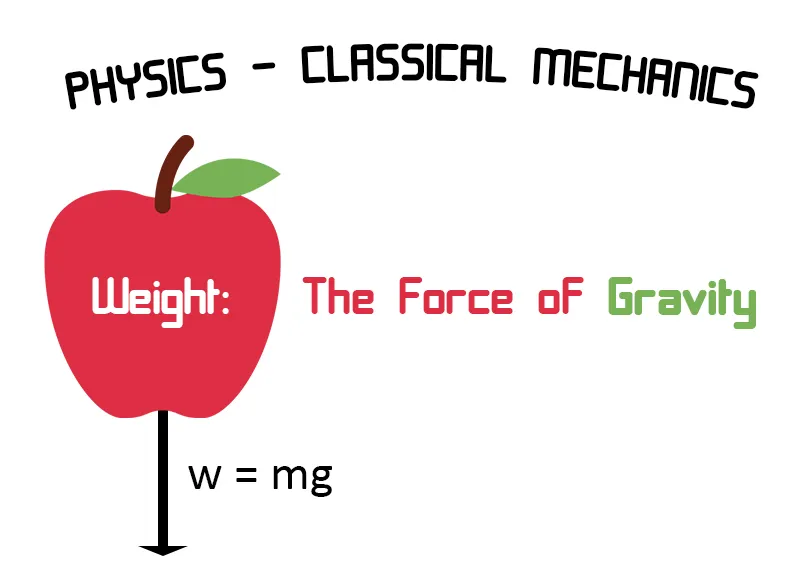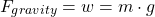Physics - Classical Mechanics - Weight: The Force of Gravity[Contains Image1]

Introduction

Hey it's a me again @drifter1! In this article we will continue with Physics, and more specifically the branch of "Classical Mechanics". Today's article will be about Weight: The Force of Gravity. So, without further ado, let's get straight into it!

Weight Force w

We already used the weight w of an object in various topics and problems of Physics.

The weight w is equal to the product of an object's mass m and the gravitational acceleration g caused by the force of gravity that attracts the object to the Earth for example.

Thus, mathematically the force of gravity can be described by the following equation:For Earth we use the accelaration of gravity on the surface of the Earth at sea level, which is: g = 9.8 m/s2.

Gravitational Acceleration g

The gravitational acceleration that an object acts on another object, can be calculated by substituting F with m ⋅ g in Newton's Law of Gravity:The mass m gets cancelled on both sides giving us an equation for calculating g.

Thinking about planets we can describe the distance d as the radius of a planet r plus the relative height of the object h to the surface of the planet, giving us:Gravity on Earth

Let's calculate the value of Earth's gravity at the Earth's surface and some Km's above the surface. Earth's mass is approx. 5.98 × 1024 Kg and the typical radius is 6.38 × 106 m.

Inserting this information in the previous equation we get:For twice the distance (d = 2 ⋅ r) we get:Gravity on Other Planets of the Solar SystemFinal words | Next up

And this is actually it for today's post!

Next time we will talk about Gravitational Fields....

See ya!Keep on drifting!
H2
H3
H4
3 columns
2 columns
1 column
Join the conversation now